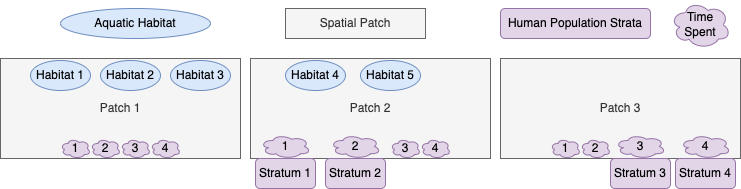library(exDE)
library(expm)
library(data.table)
library(ggplot2)
library(deSolve)

## Introduction

We show how to setup, solve, and analyze models of mosquito-borne pathogen transmission dynamics and control using modular software. This vignette is designed to explain modular notation by constructing a model with five aquatic habitats ($$l=5$$), three patches ($$p=3$$), and four human population strata ($$n=4$$). We call it 5-3-4.

### Diagram

The model 5-3-4 is designed to illustrate some important features of the framework and notation. We assume that:

• the first three habitats are found in patch 1; the last two are in patch 2; patch 3 has no habitats.

• patch 1 has no residents; patches 2 and 3 are occupied, each with two different population strata;

• Transmission among patches is modeled using the concept of time spent, which is similar to the visitation rates that have been used in other models. While the strata have a residency (i.e; a patch they spend most of their time in), each stratum allocates their time across all the habitats.## Parameters

We already know three important parameters, $$l$$, $$p$$ and $$n$$ because they are determined in the early stages of model building. The exDE package expects all parameters to be contained in a list object, containing nHabitats, nPatches, and nStrata which correspond to l, p and n.

params = make_parameters_xde()
params$nHabitats = 5 params$nPatches = 3

### Egg Dispersal Matrix

The egg dispersal matrix $$\mathcal{U}$$ is a $$l \times p$$ matrix describing how eggs laid by adult mosquitoes in a patch are allocated among the aquatic habitats in that patch. It is also attached directly to the parameters list.

$\begin{equation} {\cal U} = \left[ \begin{array}{ccccc} .7 & 0 & 0\\ .2 & 0 & 0\\ .1 & 0 & 0\\ 0 & .8 & 0\\ 0 & .2 & 0\\ \end{array} \right] \end{equation}$

xi <- matrix(c(.7, .2, .1, .8, .2), 5, 1)
params$calU <- t(calN %*% diag(as.vector(xi))) ### Aquatic Mosquito Parameters For this simulation, we use the basic competition model of larval dynamics (see more here). It requires specification of three parameters, $$\psi$$ (maturation rates), $$\phi$$ (density-independent mortality rates), and $$\theta$$ (density-dependent mortality terms), and initial conditions. The function exDE::make_parameters_L_basic does basic checking of the input parameters and returns a list with the correct class for method dispatch. The returned list is attached to the main parameter list with name Lpar. L0 <- rep(1, params$nHabitats)
psi <- rep(1/8, params$nHabitats) phi <- rep(1/8, params$nHabitats)
theta <- c(1/10, 1/20, 1/40, 1/100, 1/10)

params = make_parameters_L_basic(pars = params, psi = psi, phi = phi, theta = theta)
params = make_inits_L_basic(pars = params, L0)

We use the ODE version of the generalized Ross-Macdonald model (see more here). Part of the specification of parameters includes the construction of the mosquito dispersal matrix $$\mathcal{K}$$, and the mosquito demography matrix $$\Omega$$. Like for the aquatic parameters, we use exDE::make_parameters_MYZ_RM_ode to check parameter types and return a list with the correct class for method dispatch. We attach the returned list to the main parameter list with name MYZpar.

g <- rep(1/12, params$nPatches) sigma <- rep(1/12/2, params$nPatches)
calK <- t(matrix(
c(c(0, .6, .3),
c(.4, 0, .7),
c(.6, .4, 0)), 3, 3))
f <- rep(1/3, params$nPatches) q <- rep(0.9, params$nPatches)
nu <- c(1/3,1/3,0)
eggsPerBatch <- 30
tau <- 12
M0 <- rep(100, params$nPatches) G0 <- rep(10, params$nPatches)
Y0 <- rep(1, params$nPatches) Z0 <- rep(0, params$nPatches)

b <- rep(0.55, params$nStrata) c <- c(0.1, .02, .1, .02) params = make_parameters_X_SIS(pars = params, b = b, c = c, r = r) params = make_inits_X_SIS(params, X0) ## Simulation ### Exogenous forcing and vector control We need to specify models for exogenous forcing and vector control. We simply use the null models for both (no presence of vector control and no exogenous forcing). params = make_parameters_exogenous_null(params) params = make_parameters_vc_null(params) params = make_parameters_mi_null(params) ### Initial Conditions After the parameters for 5-3-4 have been specified, we can generate the indices for the model and attach them to the parameter list. params = make_indices(params) Now we can set the initial conditions of the model. y0 = get_inits(params) ### Numerical Solution Now we can pass the vector of initial conditions, y, our parameter list params, and the function exDE::xDE_diffeqn to the differential equation solvers in deSolve::ode to generate a numerical trajectory. The classes of Xpar, MYZpar, and Lpar in params will ensure that the right methods are invoked (dispatched) to solve your model. out <- deSolve::ode(y = y0, times = 0:365, func = xDE_diffeqn, parms = params, method = "lsoda") ### Plot Output With a small amount of data wrangling made easier by the data.table package, we can plot the output. colnames(out)[params$L_ix+1] <- paste0('L_', 1:params$nHabitats) colnames(out)[params$M_ix+1] <- paste0('M_', 1:params$nPatches) colnames(out)[params$G_ix+1] <- paste0('G_', 1:params$nPatches) colnames(out)[params$Y_ix+1] <- paste0('Y_', 1:params$nPatches) colnames(out)[params$Z_ix+1] <- paste0('Z_', 1:params$nPatches) colnames(out)[params$X_ix+1] <- paste0('X_', 1:params$nStrata) out <- out[, -c(params$Upsilon_ix+1)]

out <- as.data.table(out)
out <- melt(out, id.vars = 'time')
out[, c("Component", "Stratification") := tstrsplit(variable, '_', fixed = TRUE)]
out[, variable := NULL]

ggplot(data = out, mapping = aes(x = time, y = value, color = Stratification)) +
geom_line() +
facet_wrap(. ~ Component, scales = 'free') +
theme_bw()